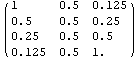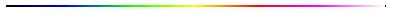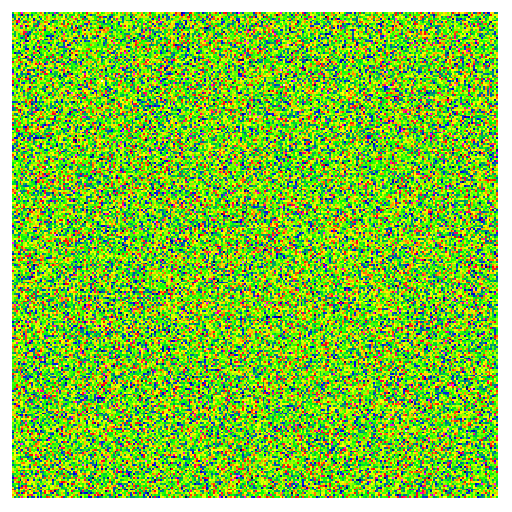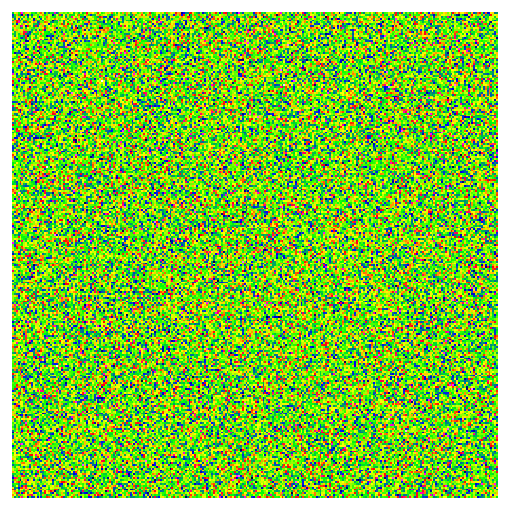Selecting Pixels from an Image Stack that Match Temporal RGB Functions

In this example, we analyze a time course of RGB data such that pixels are selected from an image stack that best fit functions of R, G, and B with respect to time.  For purposes of demonstration, the analysis is performed on a very simple image stack consisting of four RGB images representing four different times.  Pixel values are generated by a random number generator in all four frames, and then a sum-of-square-of-the-differences (SSD) measure is applied to each pixel in all time frames to determine which x,y positions in the image stack best fit a target function.  We select pixels that fit the following criteria with respect to time:

R channel - Pixel intensity decreases with a factor of two per each time (exponential decay, half-life)

G channel - Pixel intensity remains static at midrange in the grayscale

B channel - Pixel intensity increases with a factor of two per each time (exponential growth, doubling time)

The 'ideal' pixel in the stack would have the following attributes in matrix form, where R, G, and B are columns, and their values with respect to time change per row:Random pixel values are very unlikely to be so well organized in time, so we expect very few to closely fit these matrix target values.  To determine which pixels fit best, we use an SSD distance equation and then pseudocolor a resultant matrix using 'hotmap' to encode each pixel's SSD difference from the target values.Image 16.1 'Hotmap' pseudocoloring scheme used to show increasing SSD distances from left to right.

The pixel SSD distance is displayed via the pseudocoloring scheme after the minimum and maximum SSD values are determined for every pixel.  The lowest SSD value is pseudocolored as black and highest SSD value is displayed as white.  According to the hotmap pseudocolor scheme, all other SSD values are displayed after rescaling (or stretching) these values linearly between the highest and lowest SSD values - as shown below in Image 16.2.Image 16.2.  SSD distances shown in 'hot-mapped' pseudocolor for an image stack of random pixel values that were fit to criteria for R, G, and B values as a function of time.

As a general method for finding pixels that fit the target values best, we now 'clip' and discard high SSD pixels, re-stretch the SSD values of the remaining pixels through the full range of the hotmap pseudocoloring scheme, and redisplay the SSD image.  This procedure can be performed recursively so the clipping becomes more and more stringent for displaying pixels with the lowest SSD value with respect to the target matrix values.  The darker pixels, as per hotmap, are the best pixels.Image 16.3.  SSD distances displayed as an animated gif, where later frames clip high SSD values and show only those pixels that most closely fit the target function.

There are many suggestions for using this code:

1)  Use this methodology for image compression, where objects are in motion with respect to time.

2)  Acquire actual data and analyze with an appropriate fitting function:  Image a developing instant Polaroid print as a function of time on a flat bed scanner.

3) Adapt this technique to enzyme colorimetric assays run in solid phase on membranes (with due respect for US and Canadian patents assigned to Kairos Scientific Inc.) or in liquid phase in microtiter trays.  In both cases, a flatbed scanner might be more appropriate than a digital camera.  Note that the methodology in this example obviates the need for resorting data or interacting with a contour plots, and that there is a full (simultaneous) rank 4 analysis of both time and color.

4) Input hyperspectral remote sensing data.

5) Observe actual SSD values (available in the source code) and calculate corresponding probabilities to find such values as a method of testing random number generators.

6)  Modify the code to handle rank five tensors that also include temperature, such that RGB or hyperspectral data is fit according to both time and temperature.  This has thermodynamic implications for the determination of rates as a function of temperature which gives insight into activation barriers.  One interesting possibility in this category is the time course destruction of a flat panel monitor under various temperature stresses with respect to time.

7) Test error functions other than SSD.

Source code in .nb and .PDF .  Note that there is no 'hard coding' and that this source code is easily adapted for input of actual data, increasing the number of time points, increasing the number of channels, and changing the fitting function.